Civil Engineering - Highway Engineering - Discussion

Discussion :: Highway Engineering - Section 4 (Q.No.5)

5.

To compensate the loss of tractive force of vehicles along curves of radius R, the percentage reduction of gradient, is

 [A].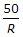[B].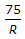[C].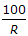[D].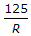[E].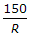Explanation:

No answer description available for this question.

 Akshay said: (Oct 28, 2018) 30r+r or 75/r whichever is minimum deducted from given gradient.

 Sushil Aryal said: (Oct 21, 2020) If the R circular curve is greater than 45m then we can use formula 75/R. Or if the R is less than 45 m then go through 30+R/R.